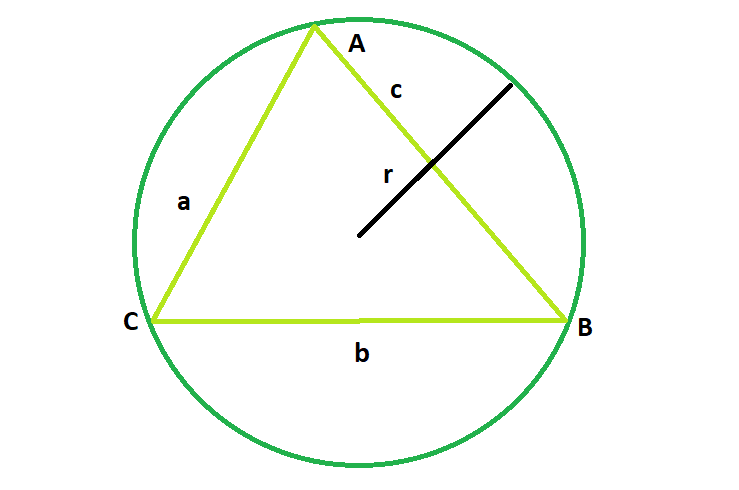GFG App
Open AppBrowser
Continue

# Area of the circumcircle of any triangles with sides given

Given a triangle with known sides a, b and c; the task is to find the area of its circumcircle.
Examples:

Input: a = 2, b = 2, c = 3
Output: 7.17714

Input: a = 4, b = 5, c = 3
Output: 19.625Approach:
For a triangle with side lengths a, b, and c,

Radius of the circumcircle:[Tex]= \frac{abc}{4\sqrt{\left ( s\left ( a+b-s \right )\left ( a+c-s \right )\left ( b+c-s \right ) \right )}}[/Tex]where A = √(s*(s-a)*(s-b)*(s-c))and s = (a+b+c)/2 is the semiperimeter.Therefore, Area of the circumcircle:Below is the implementation of the above approach:

## C++

 // C++ Program to find the area // the circumcircle of the given triangle   #include  using namespace std;   // Function to find the area // of the circumcircle float circlearea(float a, float b, float c) {       // the sides cannot be negative     if (a < 0 || b < 0 || c < 0)         return -1;       // semi-perimeter of the circle     float p = (a + b + c) / 2;       // area of triangle     float At = sqrt(p * (p - a) * (p - b) * (p - c));       // area of the circle     float A = 3.14 * pow(((a * b * c) / (4 * At)), 2);     return A; }   // Driver code int main() {       // Get the sides of the triangle     float a = 4, b = 5, c = 3;       // Find and print the area of the circumcircle     cout << circlearea(a, b, c) << endl;       return 0; }

## Java

 // Java Program to find the area // the circumcircle of the given triangle import java.*; class gfg { // Function to find the area // of the circumcircle public double circlearea(double a, double b, double c) {       // the sides cannot be negative     if (a < 0 || b < 0 || c < 0)         return -1;       // semi-perimeter of the circle     double p = (a + b + c) / 2;       // area of triangle     double At = Math.sqrt(p * (p - a) * (p - b) * (p - c));       // area of the circle     double A = 3.14 * Math.pow(((a * b * c) / (4 * At)), 2);     return A; } }   class geek { // Driver code public static void main(String[] args) {     gfg g = new gfg();     // Get the sides of the triangle     double a = 4, b = 5, c = 3;       // Find and print the area of the circumcircle     System.out.println(g.circlearea(a, b, c));   } }   //This code is contributed by shk..

## Python3

 # Python3 Program to find the area # the circumcircle of the given triangle import math   # Function to find the area # of the circumcircle def circlearea(a, b, c):       # the sides cannot be negative     if (a < 0 or b < 0 or c < 0):         return -1;       # semi-perimeter of the circle     p = (a + b + c) / 2;       # area of triangle     At = math.sqrt(p * (p - a) *                   (p - b) * (p - c));       # area of the circle     A = 3.14 * pow(((a * b * c) / (4 * At)), 2);     return A;   # Driver code   # Get the sides of the triangle a = 4; b = 5; c = 3;   # Find and print the area  # of the circumcircle print (float(circlearea(a, b, c)));   # This code is contributed  # by Shivi_Aggarwal

## C#

 // C# Program to find the area // the circumcircle of the given triangle using System; class gfg {  // Function to find the area  // of the circumcircle  public double circlearea(double a, double b, double c)  {       // the sides cannot be negative     if (a < 0 || b < 0 || c < 0)         return -1;       // semi-perimeter of the circle     double p = (a + b + c) / 2;       // area of triangle     double At = Math.Sqrt(p * (p - a) * (p - b) * (p - c));       // area of the circle     double A = 3.14 * Math.Pow(((a * b * c) / (4 * At)), 2);     return A;  } }   class geek {  // Driver code  public static int Main()  {     gfg g = new gfg();     // Get the sides of the triangle     double a = 4, b = 5, c = 3;       // Find and print the area of the circumcircle     Console.WriteLine(g.circlearea(a, b, c));       return 0;  } } //This code os contributed by SoumikMondal

## PHP

 

## Javascript

 

Output

19.625

Time Complexity: O(log(n)) as it is using inbuilt sqrt function
Auxiliary Space: O(1)

My Personal Notes arrow_drop_up